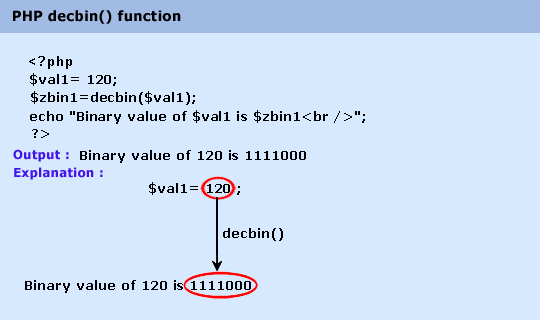# Education is not limited to just classrooms. It can be gained anytime, anywhere... - Ravi Ranjan (M.Tech-NIT)

## PHP : decbin() function

Description

The decbin() function is used to convert a decimal number to a binary number.

Version

(PHP 4 and above)

Syntax

decbin(num)

Parameter

Name

Description

Required /
Optional

Type

num

The decimal value to convert

Required

Integer

Return value

Binary string representation of num.

Value Type : String.

Pictorial PresentationExample :

1. \$val1= 120;

2. \$val2=7;

3. \$val3=9899;

4. \$zbin1=decbin(\$val1);

5. \$zbin2=decbin(\$val2);

6. \$zbin3=decbin(\$val3);

7. echo "Binary value of \$val1 is \$zbin1
";

8. echo "Binary value of \$val2 is \$zbin2
";

9. echo "Binary value of \$val3 is \$zbin3
";

10. ?>

Output :

Binary value of 120 is 1111000 Binary value of 7 is 111 Binary value of 9899 is 10011010101011